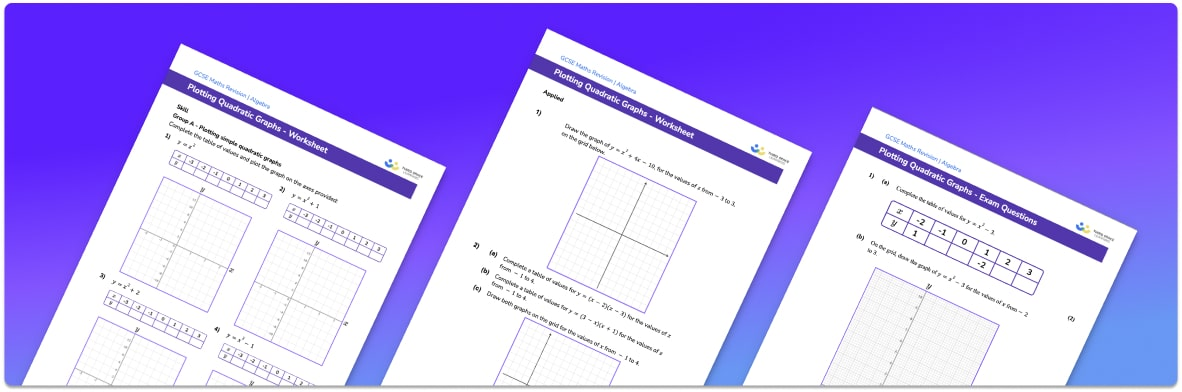• Section 1 of the plotting quadratic graphs worksheet contains 20+ skills-based plotting quadratic graphs questions, in 3 groups to support differentiation
• Section 2 contains 3 applied plotting quadratic graphs questions with a mix of worded problems and deeper problem solving questions
• Section 3 contains 3 foundation and higher level GCSE exam style plotting quadratic graphs questions
• Answers and a mark scheme for all plotting quadratic graphs questions are provided
• Questions follow variation theory with plenty of opportunities for students to work independently at their own level
• All questions created by fully qualified expert secondary maths teachers

Suitable for GCSE maths revision for AQA, OCR and Edexcel exam boards

• This field is for validation purposes and should be left unchanged.

You can unsubscribe at any time (each email we send will contain an easy way to unsubscribe). To find out more about how we use your data, see our privacy policy.

### Plotting quadratic graphs at a glance

To plot a quadratic graph we use a table of values for x and y. The required x values are often given in a question but if they are not, a reasonable range to use would be -3 to 3. The y values are then calculated using the equation of the line. For example, if we are plotting y=x^2+2 and x is 1, then y=1^2+2=3.

Once we have a table of values, we can plot the pairs of points onto a coordinate grid. Quadratic graphs should be drawn as smooth curves and the shape of the graph of a quadratic function is always a parabola. If the coefficient of x^2 is positive, the graph will be u shaped and if it is negative it will be n shaped.

For a quadratic of the form y=ax^2+bx+c, The y intercept of the graph is c. The x intercepts, or roots, of the graph can be found by solving ax^2+bx+c=0 and the vertex, or turning point of the graph, can be found by completing the square. These pieces of information can be used to make a sketch of the quadratic graph.

Quadratics and quadratic graphs feature a lot in A Level maths including finding the gradient, calculating the area under the curve, and working with imaginary numbers and so working with quadratics is an important skill to master for those wanting to progress to A Level.

Looking forward, students can then progress to additional types of graph worksheets and other algebra worksheets, for example a sequences worksheet, simultaneous equations worksheet or straight line graphs worksheet.For more teaching and learning support on Algebra our GCSE maths lessons provide step by step support for all GCSE maths concepts.

## Do you have KS4 students who need more focused attention to succeed at GCSE?There will be students in your class who require individual attention to help them succeed in their maths GCSEs. In a class of 30, it’s not always easy to provide.

Help your students feel confident with exam-style questions and the strategies they’ll need to answer them correctly with our dedicated GCSE maths revision programme.

Lessons are selected to provide support where each student needs it most, and specially-trained GCSE maths tutors adapt the pitch and pace of each lesson. This ensures a personalised revision programme that raises grades and boosts confidence.

Find out more